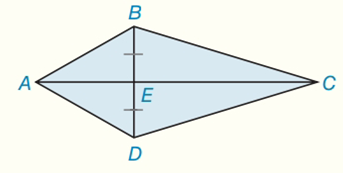Chapter 8.CR, Problem 12CRElementary Geometry For College St...

7th Edition
Alexander + 2 others
ISBN: 9781337614085

Solutions

Chapter
SectionElementary Geometry For College St...

7th Edition
Alexander + 2 others
ISBN: 9781337614085
Textbook Problem

Given: Kite ABCD with   A B = 10 B C = 17 ,  and  B D = 16 Find: A A B C DTo determine

To find:

AABCD

Explanation

Corollary:

The area A of a kite whose diagonals have lengths d1 and d2 is given by

A=12d1d2

Calculation:

The kite ABCD has lengths:

AB=10,BC=17, and BD=16

Sketch the kite ABCD as shown below:

d1=BD=16

To find d2:

AE2=AB2BE2=10282=10064=36AE=36AE=6

EC2=BC2BE2=17282

Still sussing out bartleby?

Check out a sample textbook solution.

See a sample solution

The Solution to Your Study Problems

Bartleby provides explanations to thousands of textbook problems written by our experts, many with advanced degrees!

Get Started

Solve the equations in Exercises 126. 14x2=0

Finite Mathematics and Applied Calculus (MindTap Course List)

Evaluate the definite integrals in Problems 1-32. 26.

Mathematical Applications for the Management, Life, and Social Sciences

y=4x2+1 defines y implicitly as a function of x.

Study Guide for Stewart's Single Variable Calculus: Early Transcendentals, 8th

Maximize Subject to P=3x4y x+y45x2y10x0,y0

Finite Mathematics for the Managerial, Life, and Social Sciences